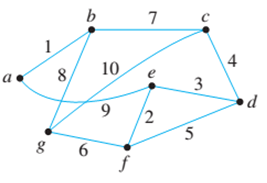Chapter 10.6, Problem 5ES### Discrete Mathematics With Applicat...

5th Edition
EPP + 1 other
ISBN: 9781337694193

#### Solutions

Chapter
Section### Discrete Mathematics With Applicat...

5th Edition
EPP + 1 other
ISBN: 9781337694193
Textbook Problem
4 views

# Use Kruskal’s algorithm to find a minimum spanning tree for each of the graphs in 5 and 6. Indicate the order in which edges are added to form each tree. 5.To determine

(a)

The minimum spanning tree for the given graph using Kruskal’s algorithm.

Explanation

Given information:

A weighted connected graph is given as,

Calculation:

Kruskal’s minimum cost spanning tree algorithm states that, keep on adding the minimum cost edge except those who create the circuit, until we reach n1 edges where n is the number of vertices in the given graph.

When we apply Kruskal’s algorithm then, edges are added in the following order

To determine

(b)

The minimum spanning tree for the given graph using Kruskal’s algorithm.

### Still sussing out bartleby?

Check out a sample textbook solution.

See a sample solution

#### The Solution to Your Study Problems

Bartleby provides explanations to thousands of textbook problems written by our experts, many with advanced degrees!

Get Started